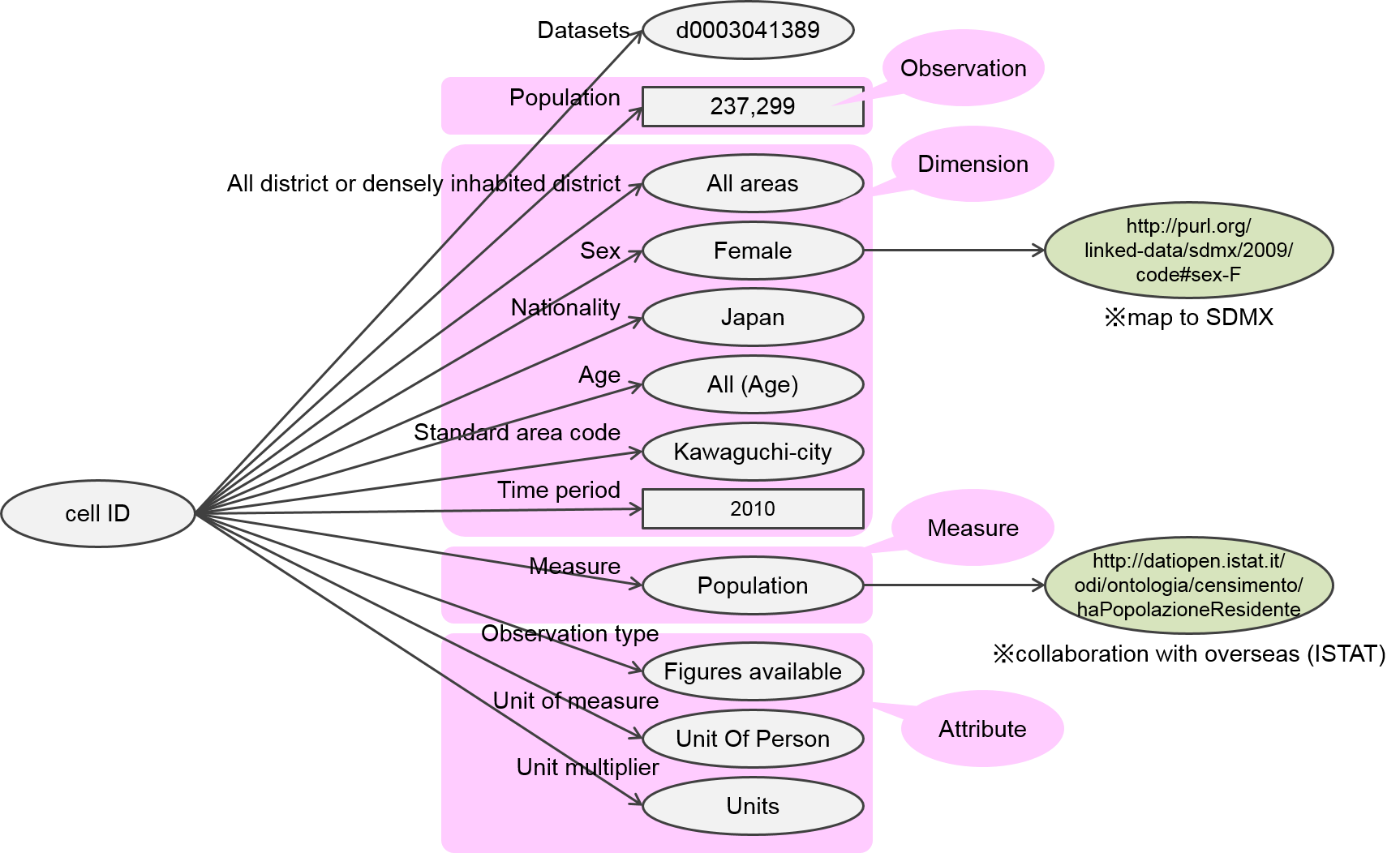# Outline

Outline of LOD and statistical LOD is explained below.

## What is LOD?

### Five stages of open data

Open data is classified in five stages by the level of openness (see "What is 5 Star Linked Data?").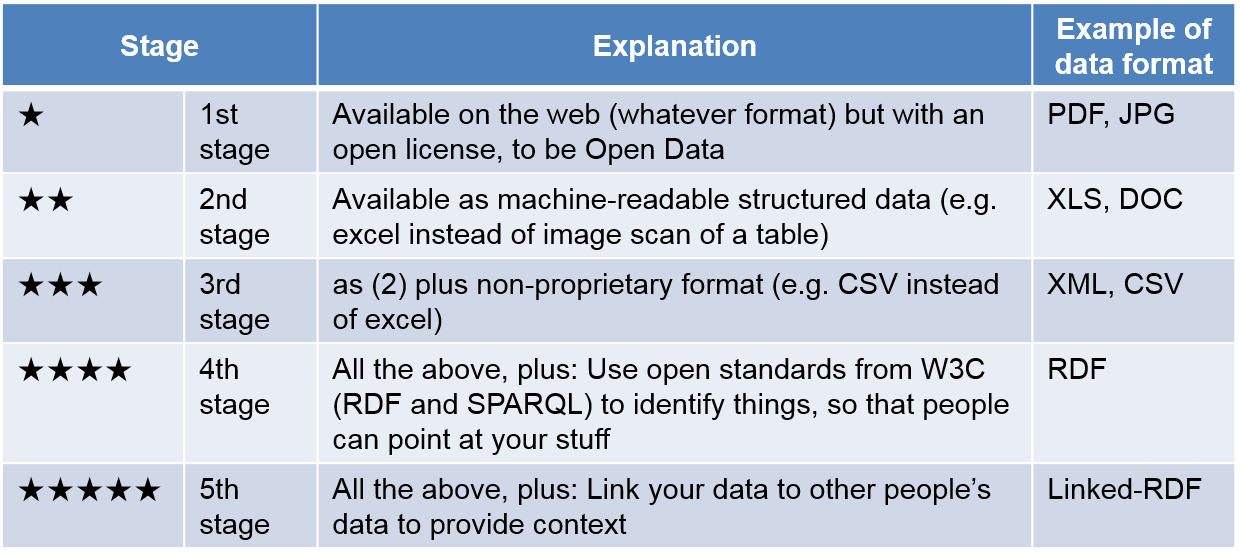In the first stage, data is available on the Web (whatever format) under an open license. The data can not be processed by computer. In the fifth stage, data can be processed by computer, entered in any other system and linked to other data. Cross site data processing can be realized easily.

e-Stat has provided statistical data by downloading PDF, Excel and csv format or by getting XML and JSON format data. The statistical LOD of Japan provides 5 star LOD.

### What can be realized by LOD?

LOD means a kind of method for opening data on Web or opened data itself. There are two major characteristics in LOD.

1) Data can be defined uniquely. The ambiguity of data can be reduced.

In LOD, each data is attached unique identifier called URI (Uniform Resource Identifier). Data can be defined uniquely by adding URI.

2) Relationship between data can be represented. Data is linked to other data.

In LOD, data is defined by using data model called RFD (Resource Description Framework). By defining relations (links) to other data, data is mutually connected and cross site retrieval can be realized easily.

## What is the statistical LOD of Japan?

### RDF of statistical data

Statistical data is defined by using RDF. RDF is a general framework and is used for various data. There is data cube model for representing statistical data. In the statistical LOD, data is defined by this model.

#### Correspondence between statistical data and cube model

According to the cube model, statistical data consists of the following four components.

• Observation : observed values (e.g. value of population, value of population ratio)
• Dimension : components that identify observations (e.g. municipality, nationality, time, sex)
• Measure : components that represent the phenomenon being observed(e.g. population, population ratio)
• Attribute : unit of measure (e.g. unit of person, percentage)#### Basic data structure of statistical data

In RDF, data is represented by three components: subject, predicate and object. For example, standard area code of Saitama-city "11100" is represented by the following structure.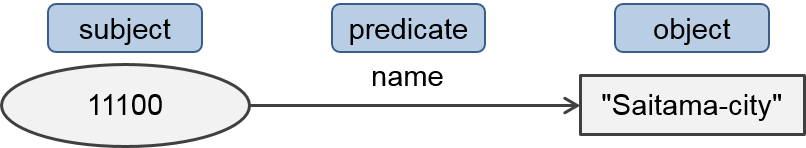For defining statistical data, each cell of statistical table is converted to RDF. The subject is ID which is assigned to each cell of statistical data. For each cell, dimensions, measure, attributes and observation value are defined as predicates and objects. One cell is represented by several triples. For example, population of kawaguchi-city age 44 male has the following data structure.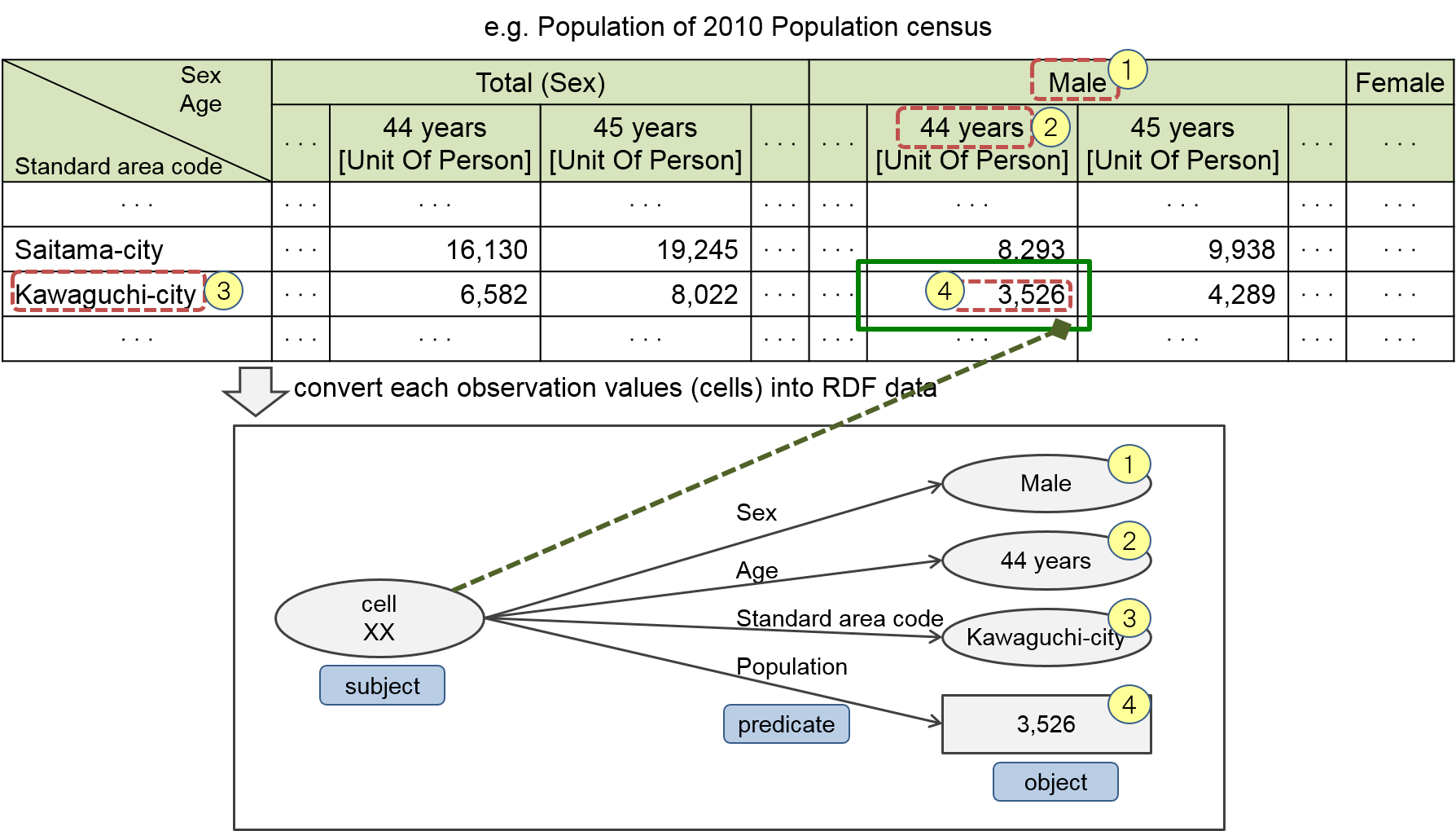#### Datasets of statistical LOD of Japan

Statistical data is classified into government statistics. Government statistics consists of statistics. Statistics consists of statistical table. In the statistical LOD of Japan, statistical table are defined as datasets.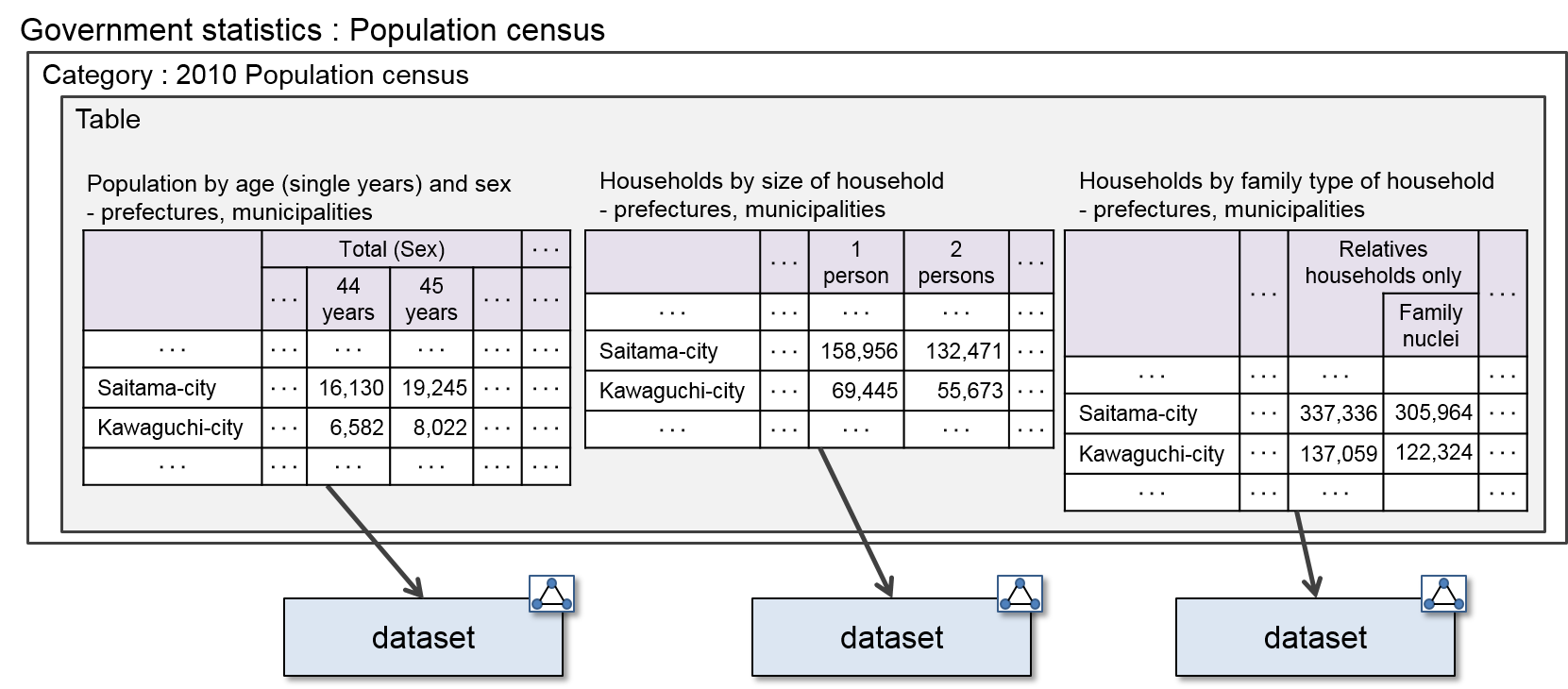For example, dataset of population census is as follows.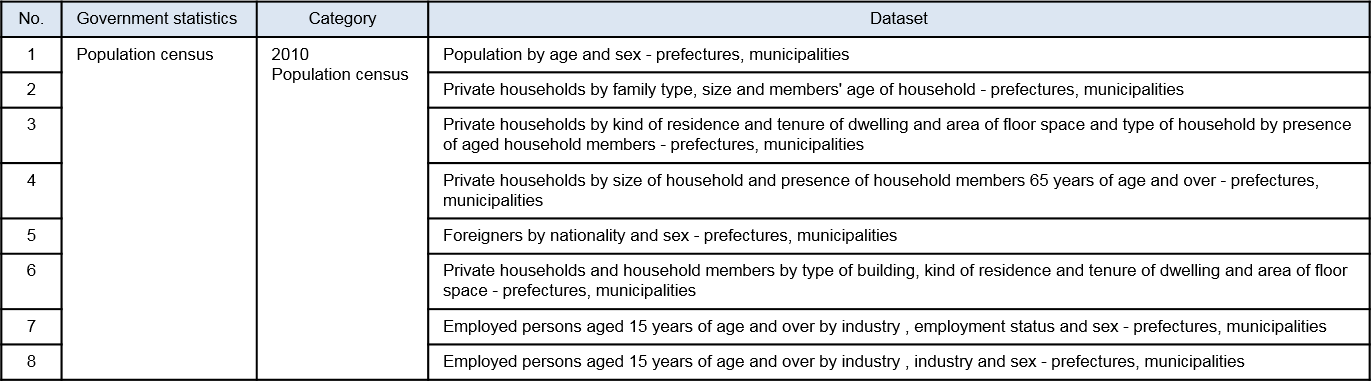### Statistical data as LOD

In LOD, RDF-defined data can be mutually linked. In the statistical LOD of Japan data is linked to vocabularies of SDMX, Istat, Eurostat and IPA.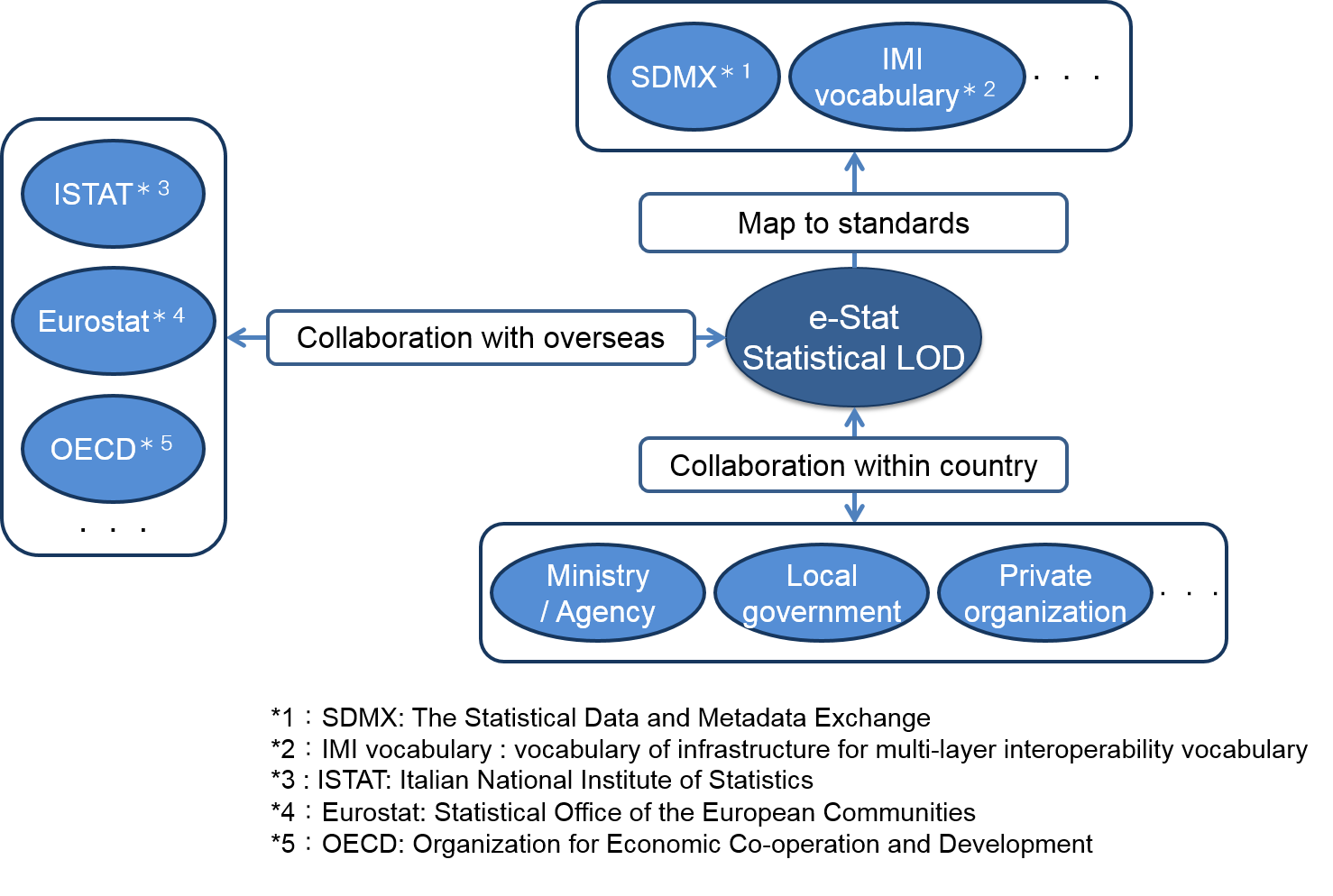### Data structure of statistical LOD of Japan

#### Concrete data structure

In the statistical LOD of Japan, each cell of statistical table is converted to RDF and linkage to outer data is defined if there is relevant data. The following illustration shows data structure for observation value.